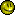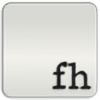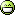Rainmeter Code Walkthrough #2 - Binary Clock
If you are unfamiliar with the binary (base 2) numeral system, you may want to do some reading here before going through the rest of this post.

The aim of this skin is to create a binary-coded decimal clock in Rainmeter.  To keep this short, we'll just do the hours and minutes (especially since the code for seconds is virtually identical to the minutes anyway).(package includes several variants - we'll be going through "Decimal_HM.ini")

### The Groundwork

To begin, we'll need [Rainmeter], [Metadata], and [Variables] sections.  Once again, we'll use include to put the variables in an external file (called Appearance.txt) so the end user can change settings easily.
`[Rainmeter]Author=Flying HyraxVersion=2001000[Metadata]Name=Binary Clock - Encoded Decimal - Hours and MinutesVersion=1.0Information=License=Creative Commons Attribution-Non Commercial-Share Alike 3.0[Variables]include=#CURRENTPATH#Appearance.txt`
(Here's the contents of Appearance.txt, for reference:)
`[Variables]Hour0=200,200,200,100Hour1=250,250,250,200Min0=200,200,200,100Min1=250,250,250,200Sec0=200,200,200,100Sec1=250,250,250,200Radius=6Space=12`
Next, back in the main file, we need Time Measures to return the "raw" time information that we will be converting into binary:
`[mHour]Measure=TIMEFormat=%H[mMin]Measure=TIMEFormat=%M`

### Math!

Now we get to the fun stuff.  For this version of the binary clock, the different place values of the decimal time each have their own column.  In other words, we'll need to convert the tens place of the hour and one's place of the hour into binary separately.  Lets say it's 1 o'clock PM (13 in the 24-hour representation) - we'll need a way to separate the "1" and the "3" in 13 from each other.  To do this, the solution I came up with is to use some Calc Measures and the FRAC and TRUNC functions.  Both functions take a number as their input. FRAC returns the fractional part (i.e. FRAC(1.234) returns .234) while TRUNC returns the whole number (TRUNC(4.321) returns 4).  Here's what we do:
`[mHourTens]Measure=CALCFormula=(TRUNC(mHour * 0.1))[mHourOnes]Measure=CALCFormula=(10 * (FRAC(mHour * 0.1)))[mMinTens]Measure=CALCFormula=(TRUNC(mMin * 0.1))[mMinOnes]Measure=CALCFormula=(10 * (FRAC(mMin * 0.1)))`
Here's what's going on there, using 13:27 as an example time.  [mHourTens] multiplies 13 by 0.1, giving 1.3, then uses TRUNC to isolate the 1.  [mHourOnes] multiplies 13 by 0.1 (gives 1.3), isolates the fractional part (0.3), then multiplies by 10 (returns 3).  [mMinTens] and [mMinOnes] do the same thing, but with the numerals in 27.  Using these Calcs, we now have the individual digits that make up the current time: 1, 3, 2, and 7.

### More math!

Now we're going to start converting those numbers into binary.  I'm sure there are several ways to do this, but for this particular skin this is the approach I took, and I think it worked rather well.  Using this method, you get one measure for each of the place values of the binary numbers, each of which returns either a 1 or a 0.  These raw numbers can then be used in a variety of different meter types.

The algorithm I used is described here, under "Short division by two with remainder."  Read that section to better understand what I'm doing in these next sections, but the TL;DR is that to convert a number from decimal to binary, you divide by two, and the remainder becomes the next least-significant bit (the next place to the left, starting at the radix point).  The quotient of the division operation is again divided by two leaving a remainder of 1 or 0; repeat until the quotient is zero.  For example, here are the Calc measures to convert the decimal ten's place into binary values:
`[mHourTensBin1]Measure=CALCFormula=(mHourTens >= 1) ? (mHourTens % 2) : 0DynamicVariables=1[mHourTensBin2]Measure=CALCFormula=(mHourTens >= 2) ? ((TRUNC(mHourTens / 2)) % 2) : 0DynamicVariables=1`
Here's the rundown.  In [mHourTenBin1], we first check if the ten's place of the decimal hour ("1" from our example above; the result of [mHourTens]) is greater than or equal to 1 using a Conditional Operator (see here also).  If the input is at least 1, we use the Modulo operation (%) to do the remainder division.

Modulo divides one number by another number, but returns the remainder of the division operation, not the quotient.  For instance, (5  % 2) would return 1, and (7 % 4) would return 3.  Especially, note that 1 % 2 returns 1, because 1 / 2 in remainder division is 0 remainder 1.  (That's pretty much the principle that makes this skin work).

[mHourTensBin2] is where things start to get fun.  Now that we've evaluated the 2^0 place using [mHourTensBin1], we need to evaluate the next least significant bit - in this case, 2^1.  Recall from here that we do this by performing remainder division on the whole integer answer from our first operation.  So what [mHourTensBin2] does, after checking that the input value is greater than or equal to 2, is divide the input by 2, TRUNC the answer to give us the whole integer, then use the Modulo operation on that.  If you are confused by my mediocre writing, it helps to use actual numbers as examples.  For instance, "2" (the ten's place if our hour is between 20 and 23):

2/2=1.0
TRUNC(1.0)=1
1%2=1

Alright then.  Next, the decimal one's place.  For the decimal ten's place there are only three possible options (0, 1, or 2), which can be represented by 2 binary places (2^0 and 2^1).  But the one's place has 10 possible options (the digits 0-9), which needs 4 binary places (2^0, 2^1, 2^2, 2^3).
`[mHourOnesBin1]Measure=CALCFormula=(mHourOnes >= 1) ? (mHourOnes % 2) : 0DynamicVariables=1[mHourOnesBin2]Measure=CALCFormula=(mHourOnes >= 2) ? ((TRUNC(mHourOnes / 2)) % 2) : 0DynamicVariables=1[mHourOnesBin4]Measure=CALCFormula=(mHourOnes >= 4) ? ((TRUNC((TRUNC(mHourOnes / 2)) / 2)) % 2) : 0DynamicVariables=1[mHourOnesBin8]Measure=CALCFormula=(mHourOnes >= 8) ? ((TRUNC((TRUNC((TRUNC(mHourOnes / 2)) / 2)) / 2)) % 2) : 0DynamicVariables=1`
This is the same process we used for the ten's place, only expanded to include up to the binary 8's (2^3) place.  The higher the place value, the longer the formula necessary to evaluate the binary value of that place, because you must do one TRUNC(n/2) for every previous place.  Hence in [mHourOneBin8] there are 3 TRUNC functions, to account for the binary 4's, 2's, and 1's places.  For instance, if [mHourOnes] was returning 9, then the process that [mHourOnesBin8] would go through would look something like this:

9/2=4.5
TRUNC(4 .5)=4
4/2=2
TRUNC(2)=2
2/2=1
TRUNC(1)=1
1 % 2 = 1

### Just a bit more math...

Next, we need to do the same thing, only for the minutes.  The ten's place for the minutes has 6 possible values (0-5), meaning we need 3 binary places; while the one's place has 10 options (0-9), so we need 4 binary places for that.
`[mMinTensBin1]Measure=CALCFormula=(mMinTens >= 1) ? (mMinTens % 2) : 0DynamicVariables=1[mMinTensBin2]Measure=CALCFormula=(mMinTens >= 2) ? ((TRUNC(mMinTens / 2)) % 2) : 0DynamicVariables=1[mMinTensBin4]Measure=CALCFormula=(mMinTens >= 4) ? ((TRUNC((TRUNC(mMinTens / 2)) / 2)) % 2) : 0DynamicVariables=1[mMinOnesBin1]Measure=CALCFormula=(mMinOnes >= 1) ? (mMinOnes % 2) : 0DynamicVariables=1[mMinOnesBin2]Measure=CALCFormula=(mMinOnes >= 2) ? ((TRUNC(mMinOnes / 2)) % 2) : 0DynamicVariables=1[mMinOnesBin4]Measure=CALCFormula=(mMinOnes >= 4) ? ((TRUNC((TRUNC(mMinOnes / 2)) / 2)) % 2) : 0DynamicVariables=1[mMinOnesBin8]Measure=CALCFormula=(mMinOnes >= 8) ? ((TRUNC((TRUNC((TRUNC(mMinOnes / 2)) / 2)) / 2)) % 2) : 0DynamicVariables=1`
...and that's all the binary conversion stuff.  To do seconds, the formulas would be the same as for the minutes - only changing all the measure names.

### Auto Scaling

Now assuming that you are still reading and that your brain isn't fried (mine sure was when I was writing this), we can move on to appearance stuff.  We'll be using Roundline meters to display our binary "1s" and "0s," and it would be nice if the end user could just change some numbers in our Appearance.txt file to make the skin whatever size they wanted.  Unfortunately, you cannot use a formula directly in a Roundline meter, so we can't just do some math in each meter to make the various pieces scale to each other.  What we can do is put the math in some more Calc measures, then plug those measures into our meters using Dynamic Variables.
`[mHourTensYPos]Measure=CALCFormula=((#Radius# * 4) + (#Space# * 3))DynamicVariables=1[mMinSecYPos]Measure=CALCFormula=((#Radius# * 2)+(#Space# * 2))DynamicVariables=1[mMinSecXPos]Measure=CALCFormula=(#Space# * 1.5)DynamicVariables=1[mSizeCalc]Measure=CALCFormula=(#Radius# * 2)[mAlways1]Measure=FREEDISKSPACETotal=1MinValue=0MaxValue=1UpdateDivider=86400`
Here's a quick diagram showing where these formulas are coming from ([mHourTensYPos], specifically):[mAlways1] is a little "cheat" measure that we plug in as the MeasureName for all of our Roundline meters - by using it, all our Roundlines will appear as solid dots.

### Meter Styles

To accomplish the "lit" vs "unlit" effect for the binary dots, I decided to use Meter Styles and Dynamic Variables.  Here's the code:
`[sHour0]LineColor=#Hour0#[sHour1]LineColor=#Hour1#[sMin0]LineColor=#Min0#[sMin1]LineColor=#Min1#[sCircle]LineWidth=1LineLength=#Radius#LineStart=0StartAngle=0RotationAngle=6.28Solid=1AntiAlias=1MeasureName=mAlways1X=rY=#Space#RW=[mSizeCalc]H=[mSizeCalc]DynamicVariables=1`
[sCircle] contains all the settings that are common to every single Roundline meter, so that we don't have to repeat them a bunch of times.  (You'll see soon how short this makes all of the Meters.)  The other Styles are used with the output of the various measures (which will be either a 1 or 0) to change which color the various meters will be, by plugging the output of a Calc measure into the "MeterStyle=" line of the matching meter as a Dynamic Variable...

### Meters

...like this:
`[HourTens2]Meter=ROUNDLINEMeterStyle=sCircle | sHour[mHourTensBin2]X=#Space#Y=[mHourTensYPos][HourTens1]Meter=ROUNDLINEMeterStyle=sCircle | sHour[mHourTensBin1][HourOnes8]Meter=ROUNDLINEMeterStyle=sCircle | sHour[mHourOnesBin8]X=#Space#RY=#Space#[HourOnes4]Meter=ROUNDLINEMeterStyle=sCircle | sHour[mHourOnesBin4][HourOnes2]Meter=ROUNDLINEMeterStyle=sCircle | sHour[mHourOnesBin2][HourOnes1]Meter=ROUNDLINEMeterStyle=sCircle | sHour[mHourOnesBin1][MinuteTens4]Meter=ROUNDLINEMeterStyle=sCircle | sMin[mMinTensBin4]X=[mMinSecXPos]RY=[mMinSecYPos][MinuteTens2]Meter=ROUNDLINEMeterStyle=sCircle | sMin[mMinTensBin2][MinuteTens1]Meter=ROUNDLINEMeterStyle=sCircle | sMin[mMinTensBin1][MinuteOnes8]Meter=ROUNDLINEMeterStyle=sCircle | sMin[mMinOnesBin8]X=#Space#RY=#Space#[MinuteOnes4]Meter=ROUNDLINEMeterStyle=sCircle | sMin[mMinOnesBin4][MinutesOnes2]Meter=ROUNDLINEMeterStyle=sCircle | sMin[mMinOnesBin2][MinuteOnes1]Meter=ROUNDLINEMeterStyle=sCircle | sMin[mMinOnesBin1][spacer]Meter=IMAGESolidColor=0,0,0,1X=RY=RW=#Space#H=#Space#`
As you can see, all the Roundline meters share sCircle, but depending on whether or not the meter is for the hours or minutes, and whether or not the Measure for the appropriate binary place returns a 1 or 0, the second style is either sHour0, sHour1, sMin0, or sMin1; and this second style contains the LineColor for the Roundline meter.  The last meter is just an empty box with the dimensions of our #Space# variable, so that when the skin snaps to the edges of the screen or other skins, there is a gap between the circles and the edge.

That's pretty much it.  The difficulty with this skin is definitely the Calc measures used to change the Time measures into individual binary digits.  Except for that, the rest of the skin is fairly straightforward.

-fhI'm an idiot. I think I need a youtube video, but I can't find one. Oh well, after I finish my first class I'll come back to this.thanks. i am new in rainmeter so this is very useful.Thanks a lot man, I'm a complete newbie at this so I learnt so much from your post. Hopefully I can start to develop my own skins nowCool, I'm glad it was helpful.Good luck writing your skins!Thank you for the details, it helped me a lot for the skin I'm developing!Cool, I'm glad I could help!Bartcore3Hobbyist General Artist
It's sad to see no comments on this. You've probably put alot of work in this walkthrough!
Thank you!Hey, thanks for the comment!It was a bit of work, but I like writing, and explaining things actually helps me understand my own code a lot better.Bartcore3Hobbyist General Artist
indeedteaching is the best way to learn it yourself!